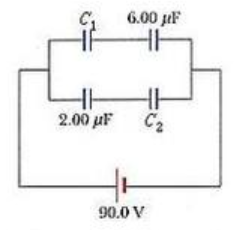# Problem: Consider the system of capacitors shown in the figure below (C1 = 2.00 μF, C2 = 4.00 μF).(a) Find the equivalent capacitance of the system.(b) Find the charge on each capacitor.(c) Find the potential difference across each capacitor.(d) Find the total energy stored by the group.

###### FREE Expert Solution

For 2 capacitors in series, the equivalent capacitance is:

$\overline{){{\mathbf{C}}}_{\mathbf{e}\mathbf{q}}{\mathbf{=}}\frac{{\mathbf{C}}_{\mathbf{1}}{\mathbf{C}}_{\mathbf{2}}}{{\mathbf{C}}_{\mathbf{1}}\mathbf{+}{\mathbf{C}}_{\mathbf{2}}}}$

For capacitors in parallel, the equivalent capacitance is:

$\overline{){{\mathbf{C}}}_{\mathbf{e}\mathbf{q}}{\mathbf{=}}{{\mathbf{C}}}_{{\mathbf{1}}}{\mathbf{+}}{{\mathbf{C}}}_{{\mathbf{2}}}{\mathbf{+}}{\mathbf{.}}{\mathbf{.}}{\mathbf{.}}{\mathbf{+}}{{\mathbf{C}}}_{{\mathbf{n}}}}$

The charge stored by a capacitor:

$\overline{){\mathbf{Q}}{\mathbf{=}}{\mathbf{C}}{\mathbf{V}}}$

(a)

Capacitors C1 and 6.0μF are in series.

Therefore, their equivalent capacitance is:

(C1)(6.00)(C1 + 6.00) = (2.00)(6.00)/(2.00 + 6.00) = 1.50 μF

Similarly, 2.00μF and C2 are in series.

87% (381 ratings)###### Problem Details

Consider the system of capacitors shown in the figure below (C1 = 2.00 μF, C2 = 4.00 μF).(a) Find the equivalent capacitance of the system.

(b) Find the charge on each capacitor.

(c) Find the potential difference across each capacitor.

(d) Find the total energy stored by the group.